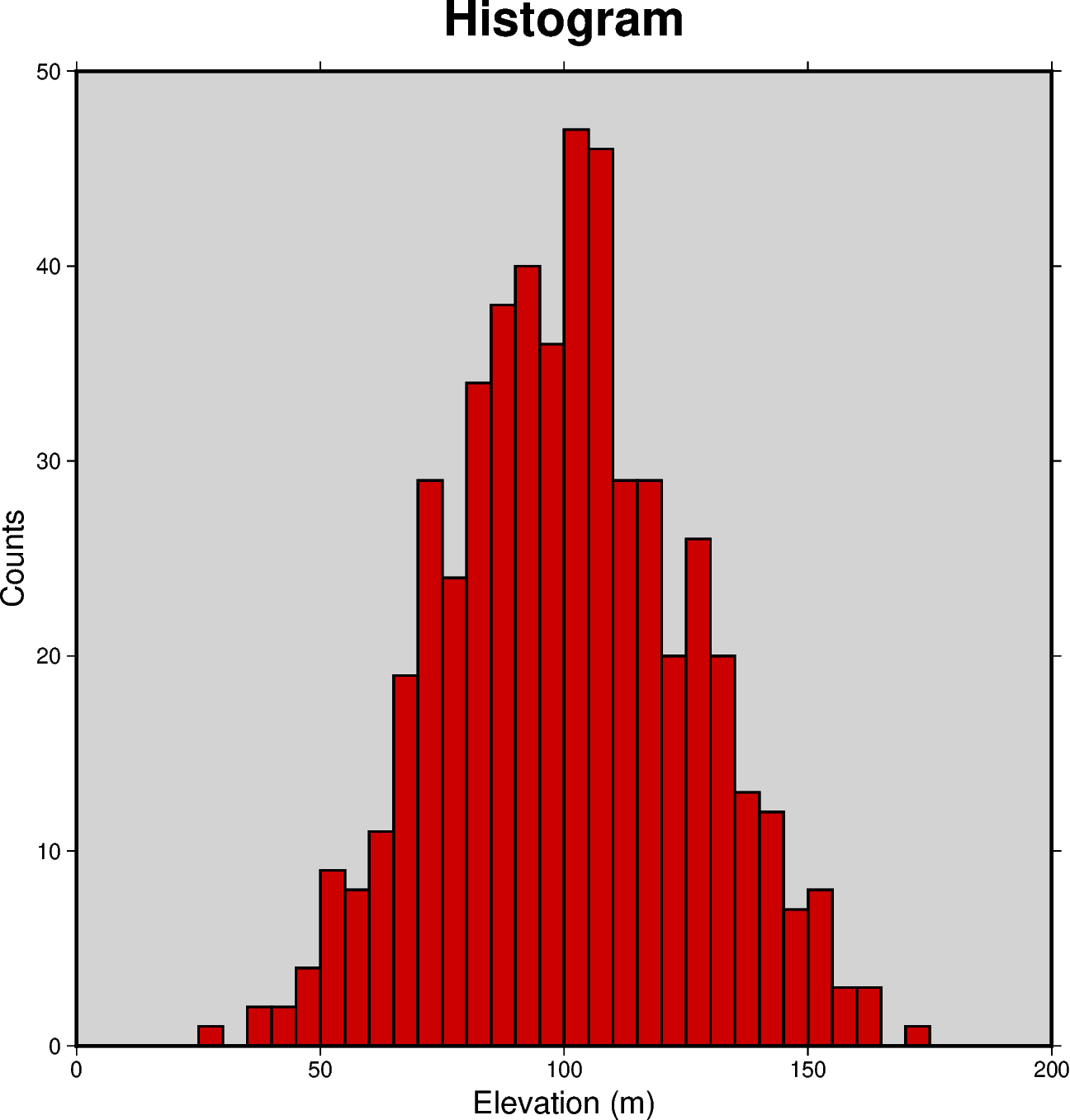# Histogram

The `pygmt.Figure.histogram` method can plot regular histograms. Using the `series` parameter allows to set the interval for the width of each bar. The type of histogram (frequency count or percentage) can be selected via the `histtype` parameter.Out:

```<IPython.core.display.Image object>
```

```import numpy as np
import pygmt

np.random.seed(100)

# generate random elevation data from a normal distribution
mean = 100  # mean of distribution
stddev = 25  # standard deviation of distribution
data = mean + stddev * np.random.randn(521)

fig = pygmt.Figure()

fig.histogram(
data=data,
# define the frame, add title and set background color to
# lightgray, add annotations for x and y axis
frame=['WSne+t"Histogram"+glightgray', 'x+l"Elevation (m)"', 'y+l"Counts"'],
# generate evenly spaced bins by increments of 5
series=5,
# use red3 as color fill for the bars
fill="red3",
# use a pen size of 1p to draw the outlines
pen="1p",
# choose histogram type 0 = counts [default]
histtype=0,
)

fig.show()
```

Total running time of the script: ( 0 minutes 1.001 seconds)

Gallery generated by Sphinx-Gallery